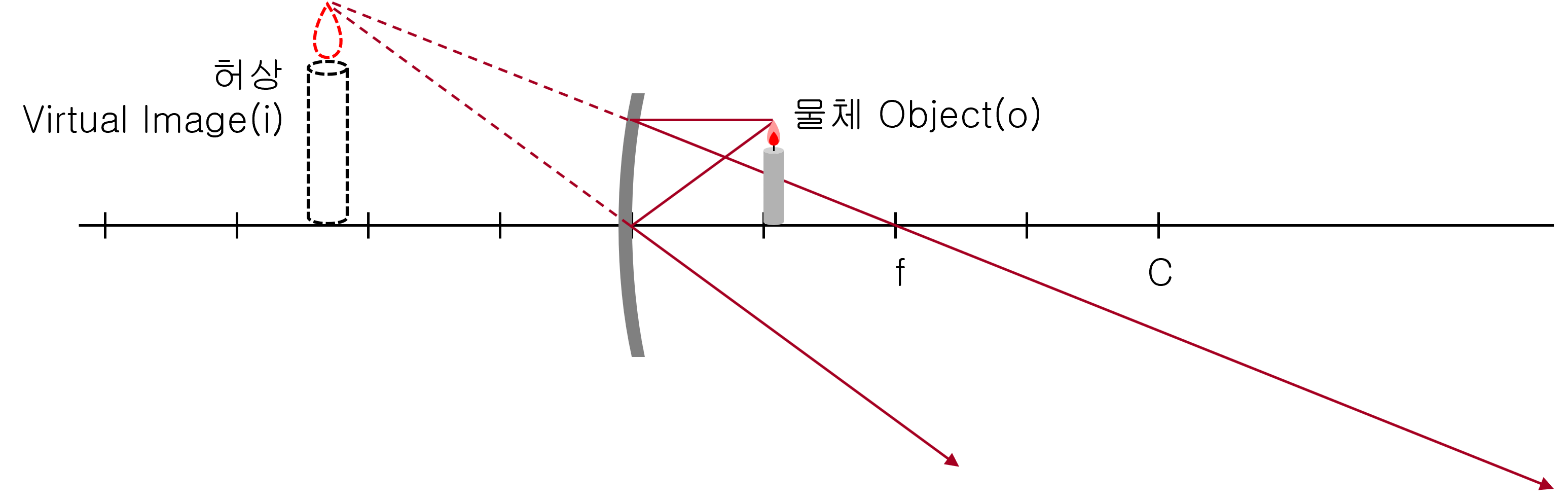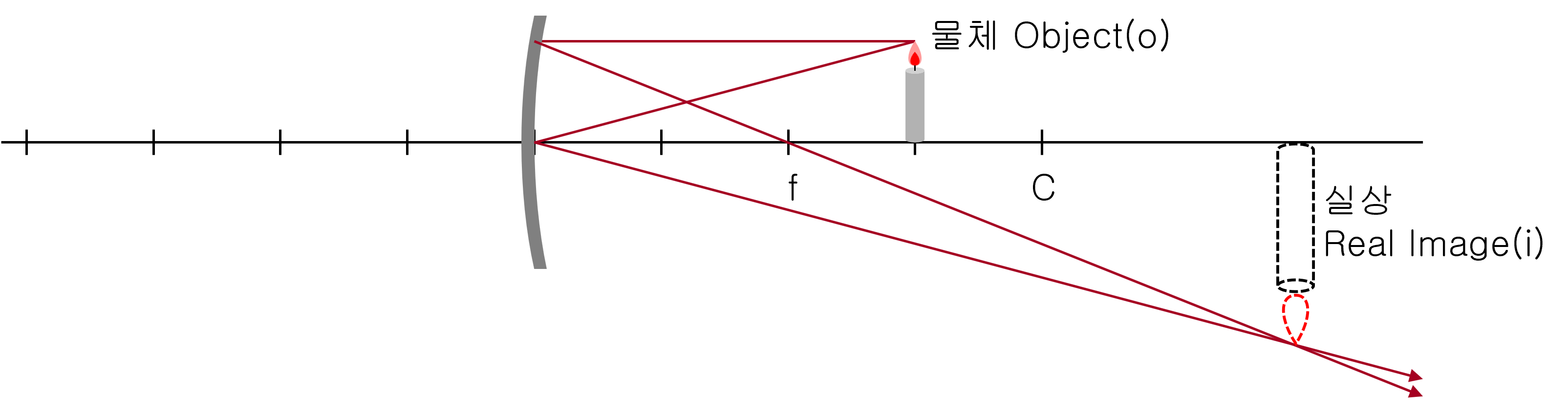# Convex Mirror & Concave Mirror

## Real image and virtual image

Looking at an object, we feel there is an object in it. By the way, if you feel that there is something, we say there is an ‘image’, even if there is no real object or emitter.
The ‘image’ in optics is caused by the refraction or reflection of light.

Real image: Indicates that the light is actually gathered there and then starts again. If you put paper in the position where the actual image is formed, you can actually see the image formed on the paper.

Virtual image: A case where there is no light at the place where ‘image’ is formed. If you put paper in the position where the image is formed, no image appears.

## A virtual image of a convex mirror

The convex mirror image is always a virtual image, standing like an object, and looks smaller than the real thing. It is used in car rearview mirrors and surveillance mirrors because it can be seen in a wide range.## Real image and virtual image of concave mirror

The concave mirror differs greatly depending on whether the object is inside the focus of the concave mirror.

If the object is in the focus of the mirror: It is a virtual image, the image looks larger than the real thing. And the image always stands straight.If the object is outside the focus of the mirror: It is a real image, which is enlarged or reduced by the distance from the mirror. And it is always reversed.The inside of the spoon can be seen as a concave mirror and the outside can be seen as a convex mirror.

## Getting the distance of the image

The following simple formula is established between the distance of the object from the mirror ‘p,’ the distance of the image from the mirror ‘i’, and the focal length of the mirror ‘f.’

$\frac { 1 }{ o } +\frac { 1 }{ i } =\frac { 1 }{ f } =\frac { 2 }{ C }$

• o: Distance from mirror to object. It always has positive (+) value.
• i: Distance from mirror to image
• f: Focal length
• C: Center of curvature, about twice the focal length

In the case of a convex mirror

• o > 0: Real object
• i < 0: Virtual image
• f < 0: Virtual focus

In the case of a concave mirror

• o > 0: Real object
• f > 0: Real focus
• i = ?: It depends on the situation.
• In the case of o < f : i < 0 (virtual image standing upright)
• In the case of o = f : i value can not be obtained.
• In the case of o > f : i < 0 (Inverted real image)

## Ray tracing method

If you trace the path of at least two rays, you can find the image.

1. A ray parallel to the central axis passes through the focus after reflection.
2. A ray that passes through the focus and enters the mirror is reflected and travels parallel to the axis.
3. A ray that passes through the center of curvature C and enters the mirror will step back through the incident path.
4. The light rays entering the center of the mirror are reflected at symmetrical angles. (General law of reflection)

## Obtaining Magnification

The magnification of the image is…

$m=-\frac { i }{ o }$

• m: magnification
• i: Distance from mirror to image
• o: Distance from mirror to object

Depending on the value of m calculated, the image looks like this:

• m > 0: The image stands straight.
• m < 0: The image is inverted.
• |m| > 1: The image is magnified.
• |m| < 1: The image is reduced.